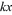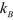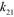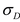Four state ratchet model

The essential features of a minimal strain-dependent ratchet model of muscle crossbridge action are: (1) dynamic binding of the S1-products complex to actin through a disorder-order transition coupled to the release of inorganic phosphate; (2) the absence of a force-generating rotation of the myosin head between the two force-holding states A.M.ADP and A.M; (3) strain-control of ADP release and ATP binding; (4) the response to large perturbations is often sensitive to the number of actin sites used, and to the inclusion of a 1 nm displacement of the neck region on release of ADP.

The kinetics and some structural aspects of the actomyosin ratchet model are defined by only four states: M.ADP.Pi, A.M.ADP, A.M and M.ATP, and where, for convenience, state are denoted by numeric labels 1-4, respectively. The attached states A.M.ADP and A.M carry tension     generated by elastic strain in a crossbridge.A minimal actin-myosin crossbridge includes: the M.ATP and M.ADP.Pi states are normally detached but may include weakly bound A-states of variable angle and zero stiffness. They produce no force. The A.M.ADP and A.M. states are strongly bound with the same conformation and the same tension       . Here    is crossbridge stiffness, and     is change of crossbridge length from zero force crossbridge length.The model is formally defined by the actin-S1 myosin cycle (see Figure) and expressions for strain-dependent rate transition rates the four state ratchet model (Smith, 1998) are:

1. For binding M.ADP.Pi      A.M.ADP the activation energy is developed as a polynomial in    , giving forward/ backward rates:where    is crossbridge stiffness,    is absolute temperature and      is Boltzmann’s constant. The polynomial coefficients        and rate constants       and         are the strain-free rates. Because this transition consists of two steps (i.e., binding to actin and power stroke), accordingly the activation energy is also supplied by two processes: i) during binding to actin thermally through Brownian fluctuations either in S1 position along the filament axis or its orientation, depending whether the elastic element lies in the S2 tail or in distal neck of each S1; ii) by release of phosphate from the A-state that provides a substantial drop in Gibbs energy, which is assumed to be part of the disorder-to-order process (i.e., A to R transition, therefore, power stroke). The backward rate,       , can be calculated from the equilibrium constant                                               .2. For strain-dependent ADP release (i.e., A.M.ADP      A.M) the difference between initial and final strain energies gives the forward rates as:where    is displacement that lever arm must move to open the nucleotide pocket and allow ADP release,      is dimensionless quantity related to the stiffness of the nucleotide binding pocket, and         strain free rate constant. The backward rate,         , can be calculated from the equilibrium constant                                           .3. Similarly, the rate constant that involve ATP binding and detaching myosin from actin (i.e., A.M       M.ADT) is:where        is displacement that lever arm must move backward after ATP binding to myosin, dissociate from actin filament and hydrolyses ATP to ADP and Pi,      is dimensionless quantity related to the stiffness of the pocket, and         is train free rate constant. The backward rate is very small and taken to be equal to zero.

4. The hydrolysis step M.ATP      M.ADT.Pi  is strain independent and is defined by         rates         and .